Get our FREE VBA eBook of the 30 most useful Excel VBA macros.

Automate Excel so that you can save time and stop doing the jobs a trained monkey could do.

# Running total in an Excel TableTables are one of the best features of Excel. While it is possible to use the standard cell referencing with a Table, they have their own referencing style called structured references. We have to think a little differently to create a running total in an Excel Table using structured references.

We will look at all the options in this post.

I recommend you download the example file for this post. Then you’ll be able to work along with examples and see the solution in action, plus the file will be helpful for future reference.

Watch the video

Contents

## The example

All the scenarios in this post use the same Table. The goal is to:

• Create a running total in the Running Total column
• Ensure values calculate correctly when rows are added or deleted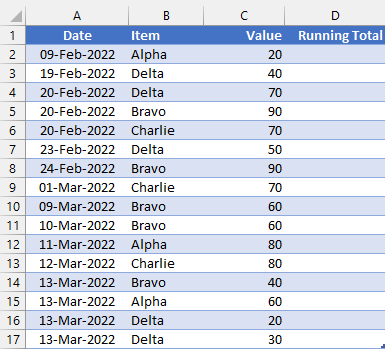## Normal cell references

We could use normal cell references in either the A1 or R1C1 style. For this, there are two common options (1) Cell above + value (2) Expanding range with mixed references.

### Method #1: Cell above + value

The purpose of a Table is to use the same formula in each row of the column. Therefore, it is not possible to just use the cell above plus the value method.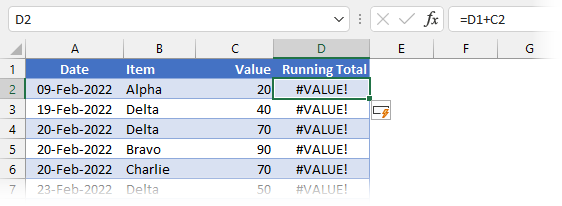The formula in Cell D2 is:

`=D1+C2`

The result of the formula is #VALUE!; Cell D1 is a text value, which cannot be added to a number using a basic calculation.

However, the SUM function ignores text values; therefore, by using SUM with a comma (known as the Union Operator), the text value will be calculated as a zero. This avoids the #VALUE! error.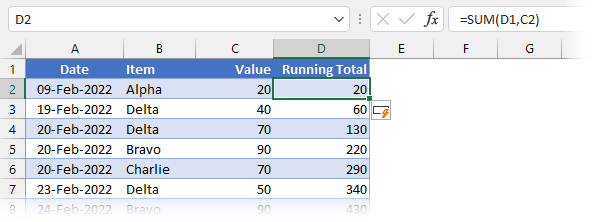The formula in Cell D2 is:

`=SUM(D1,C2)`

The result of this formula is a running total in each row of the Running Total column.

Rather than SUM, you could also use the N function. This returns zero if the cell reference within it is not a number; otherwise, it returns the number. For example, if Cell D2 contained the following formula, it would also create a running total.

`=N(D1)+C2`

However, both methods all fall short when rows are deleted. The screenshot below shows the result after Row 4 has been deleted.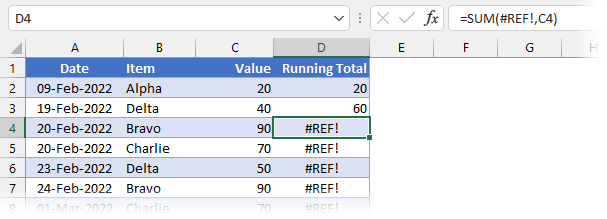As you can see by the #REF! error, this method does not work when rows are deleted. This does not meet the requirements we wanted.

### Method #2: Expanding range with mixed references

There is another method we can take using standard ranges that uses mixed references.The formula in Cell D2 is:

`=SUM(\$C\$2:C2))`

This method creates an expanding range for each row in the Table.

So, if you delete a row, it still works… perfect! Or is it? The problem comes when you add a new row of data to the bottom: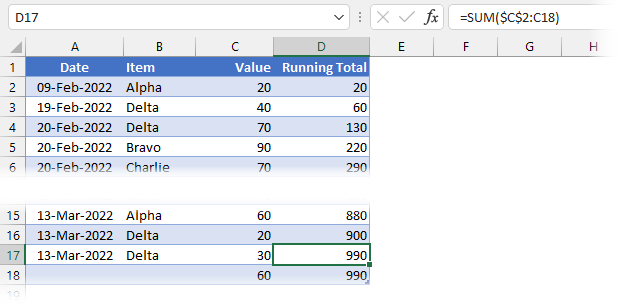There isn’t an obvious problem at the start. But, take a look at cell D17; what is the formula that has been automatically copied down? The formula references cell C18 (the last row of the Table), which is incorrect.

`=SUM(\$C\$2:C18)`

Using this method, adding rows causes the formulas to expand to include the last row, which creates a calculation issue. Therefore, this isn’t a suitable method either.## Structured references

We really want to use structured references. After all, that is the whole point of using a Table.

### Method #3: SUM with header row

In the previous section, we identified that SUM ignores text values. Given that the header row in a Table is always text and an absolute row reference (i.e., it doesn’t move when a cell is dragged down), we can create a running total with just the SUM function and structured references.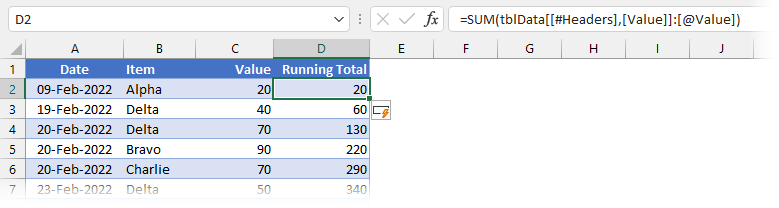The formula in Cell D2 is:

`=SUM(tblData[[#Headers],[Value]]:[@Value])`

The result of this formula is a running total in each row.

• tblData[[#Headers],[Value]] is the reference to the header row
• [@Value] is the reference to the cell within the Value column contained on the same row
• : (Colon) between two cell references creates a range

It is easier to see this method at work when we select a cell from the Running Total column.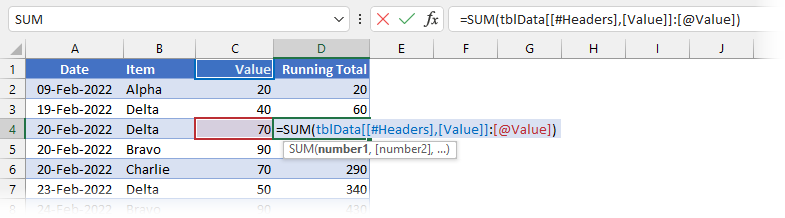Even though the entire range is not shown, a single range is created between the blue and red boxes.

We can delete or add to the Table, and it still works. AMAZING!

This appears to be the perfect method. However, I feel that using the header row makes this more of a visual solution than a data solution. By which I mean, in other parts of Excel, such as Power Query and Power Pivot, it’s not possible to reference the value in a header row. Therefore, this only works because the data is contained on an Excel worksheet.

I know this works, but conceptually, I feel we should look for another solution.

### Method #4: INDEX function

All is not lost; there is another method.

The INDEX function makes it possible to reference any cell within a column.

`=INDEX([Value],1)`

Therefore, rather than using the column header cell, we can create an absolute reference by selecting the first cell in the column using the INDEX function.

Look at the solution below: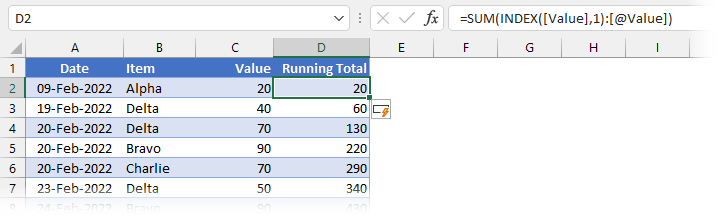The formula in Cell D2 is:

`=SUM(INDEX([Value],1):[@Value])`
• INDEX([Value],1) is always a reference to the first cell in the Value column
• [@Value] is the reference to the cell within the Value column contained on the same row
• : (Colon) between the two cell references creates a range

The result of this formula is a non-volatile running total in each row of the Running Total column. It calculates correctly even if rows are added or deleted.

Perfect! This is my preferred option.

## Other options

There are other options using OFFSET and relative named ranges that we could use. However, I have discounted these because:

• OFFSET is a volatile function that recalculates every time a cell changes in Excel, which can cause slow spreadsheet calculation times.
• Relative named ranges are a technique to use when you’re stuck or backed into a corner. However, we have suitable techniques without resorting to this.

## Running total with criteria

Let’s suggest that we don’t want a single running total, but the running total for each individual element in the Item column. Can our INDEX method handle that situation? You bet it can.

### Method #5: INDEX with SUMIFS

We will use the same INDEX technique inside the SUMIFS function.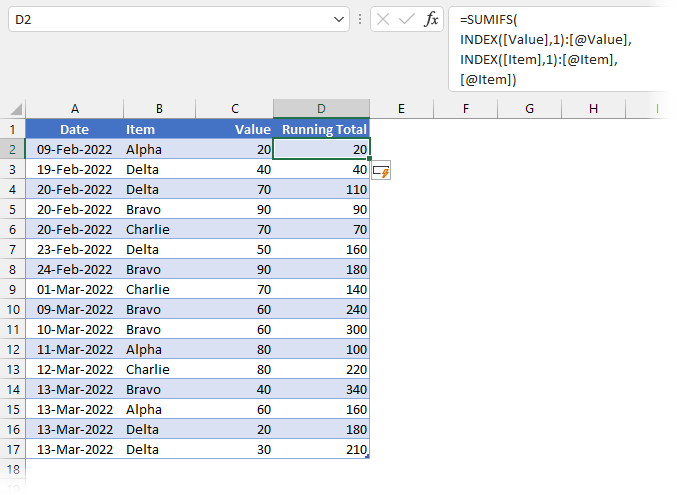The formula in Cell D2 is:

`=SUMIFS(INDEX([Value],1):[@Value],INDEX([Item],1):[@Item],[@Item])`

You will notice this uses the same INDEX method as above to create a dynamic range inside both the sum_range and criteria_range1 arguments of the SUMIFS function.

Let’s check this works:

• Alpha exists in Cells B2 (20) and B12 (80). The value in Cell C12 should therefore be 100, which it is. ✅
• Alpha also exists in Cell B15 (60). The value in Cell C15 should be 160, which it is.✅

This method works when rows and added or deleted, precisely what we need. See, the INDEX method is very flexible.

## Conclusion

There you have it, lots of options to achieve a running total in an Excel Table. But, there are also lots of pitfalls.

The methods using standard references cannot guarantee the correct values when adding or deleting rows, so these should be discounted.

Both structured referencing methods can be used with COUNT, COUNTIFS, MIN, MINIFS, MAX, MAXIFS, AVERAGE, and AVERAGEIFS.

The SUM + header row method works, but conceptually, I have an issue with referencing the header row in this way. Don’t get me wrong; if it were the only option, I would definitely use it.

The SUM + INDEX method is my preferred choice. It’s flexible and provides everything we need.Get our FREE VBA eBook of the 30 most useful Excel VBA macros.

Automate Excel so that you can save time and stop doing the jobs a trained monkey could do.

Don’t forget:

If you’ve found this post useful, or if you have a better approach, then please leave a comment below.

Do you need help adapting this to your needs?

I’m guessing the examples in this post didn’t exactly meet your situation.  We all use Excel differently, so it’s impossible to write a post that will meet everybody’s needs.  By taking the time to understand the techniques and principles in this post (and elsewhere on this site) you should be able to adapt it to your needs.

But, if you’re still struggling you should:

1. Read other blogs, or watch YouTube videos on the same topic.  You will benefit much more by discovering your own solutions.
2. Ask the ‘Excel Ninja’ in your office.  It’s amazing what things other people know.
3. Ask a question in a forum like Mr Excel, or the Microsoft Answers Community.  Remember, the people on these forums are generally giving their time for free.  So take care to craft your question, make sure it’s clear and concise.  List all the things you’ve tried, and provide screenshots, code segments and example workbooks.
4. Use Excel Rescue, who are my consultancy partner.   They help by providing solutions to smaller Excel problems.

What next?
Don’t go yet, there is plenty more to learn on Excel Off The Grid.  Check out the latest posts:

## 2 thoughts on “Running total in an Excel Table”

1.Jon Peltier says:

I’ve used a Carlson of Method 1:
=SUM(OFFSET(D1,-1,0),C2)
But lately I prefer Method 4 with INDEX.

•Excel Off The Grid says:

OFFSET is another good option. There is always the volatile function risk, but if managed correctly (which I’m sure you would), it’s not a problem.

INDEX is my “go to” for almost everything. This can also cause problems, as often miss the easier alternative. 😁